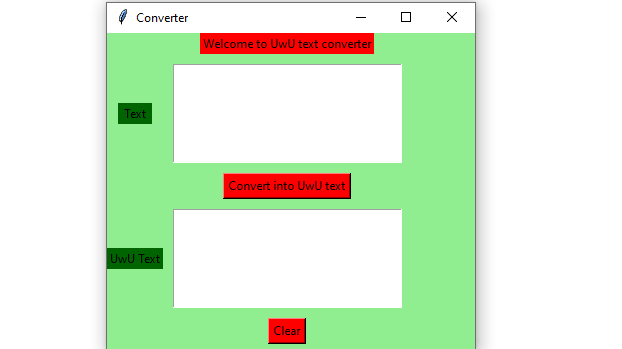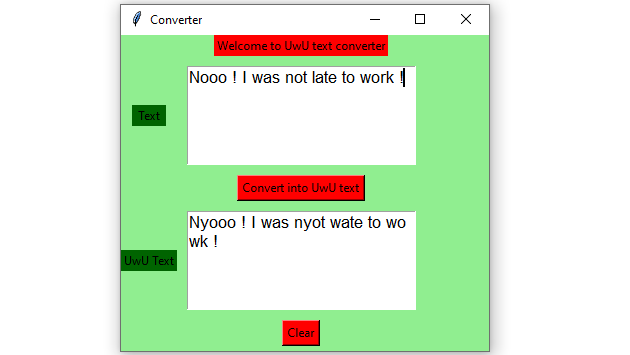# Python – UwU text convertor GUI using Tkinter

Prerequisites :Introduction to tkinter | UwU text convertor

Python offers multiple options for developing a GUI (Graphical User Interface). Out of all the GUI methods, tkinter is the most commonly used method. It is a standard Python interface to the Tk GUI toolkit shipped with Python. Python with tkinter outputs the fastest and easiest way to create GUI applications. Now, it’s up to the imagination or necessity of a developer, what he/she wants to develop using this toolkit.

To create a tkinter :

• Importing the module – tkinter
• Create the main window (container)
• Add any number of widgets to the main window.
• Apply the event Trigger on the widgets.

Let’s create a GUI based uwu-text-convertor application that can convert text input given by user into uwu text.

Below is the implementation :

 `# import all functions from the tkinter   ` `from` `tkinter ``import` `*` ` `  `# Function to clear both the text areas ` `def` `clearAll() : ` `    ``# whole content of text area  is deleted  ` `    ``text1_field.delete(``1.0``, END) ` `    ``text2_field.delete(``1.0``, END) ` ` `  `# Function to convert into UwU text  ` `def` `convert() : ` ` `  `    ``# get a whole input content from text box ` `    ``# ignoring \n from the text box content ` `    ``input_text ``=` `text1_field.get(``"1.0"``, ``"end"``)[:``-``1``] ` `     `  `    ``# the length of the input text  ` `    ``length ``=` `len``(input_text)  ` `       `  `    ``# variable declaration for the output text  ` `    ``output_text ``=` `''  ` `       `  `    ``# check the cases for every individual character  ` `    ``for` `i ``in` `range``(length):  ` `           `  `        ``# initialize the variables  ` `        ``current_char ``=` `input_text[i]  ` `        ``previous_char ``=` `None` `           `  `        ``# assign the value of previous_char  ` `        ``if` `i > ``0``:  ` `            ``previous_char ``=` `input_text[i ``-` `1``]  ` `           `  `        ``# change 'L' and 'R' to 'W'  ` `        ``if` `current_char ``=``=` `'L'` `or` `current_char ``=``=` `'R'``:  ` `            ``output_text ``+``=` `'W'` `           `  `        ``# change 'l' and 'r' to 'w'  ` `        ``elif` `current_char ``=``=` `'l'` `or` `current_char ``=``=` `'r'``:  ` `            ``output_text ``+``=` `'w'` `           `  `        ``# if the current character is 'o' or 'O'  ` `        ``# also check the previous charatcer  ` `        ``elif` `current_char ``=``=` `'O'` `or` `current_char ``=``=` `'o'``:  ` `            ``if` `previous_char ``=``=` `'N'` `or` `previous_char ``=``=` `'n'` `or` `previous_char ``=``=` `'M'` `or` `previous_char ``=``=` `'m'``:  ` `                ``output_text ``+``=` `"yo"` `            ``else``:  ` `                ``output_text ``+``=` `current_char  ` `           `  `        ``# if no case match, write it as it is  ` `        ``else``:  ` `            ``output_text ``+``=` `current_char  ` `   `  `    ``text2_field.insert(``'end -1 chars'``, output_text) ` ` `  ` `  `# Driver code  ` `if` `__name__ ``=``=` `"__main__"` `:  ` ` `  `    ``# Create a GUI window  ` `    ``root ``=` `Tk() ` ` `  `    ``# Set the background colour of GUI window   ` `    ``root.configure(background ``=` `'light green'``)   ` `     `  `    ``# Set the configuration of GUI window (WidthxHeight)  ` `    ``root.geometry(``"400x350"``)   ` ` `  `    ``# set the name of tkinter GUI window   ` `    ``root.title(``"Converter"``) ` `     `  `    ``# Create Welcome to Morse Code Translator label   ` `    ``headlabel ``=` `Label(root, text ``=` `'Welcome to UwU text converter'``,   ` `                      ``fg ``=` `'black'``, bg ``=` `"red"``)   ` `   `  `    ``# Create a "Text " label   ` `    ``label1 ``=` `Label(root, text ``=` `" Text "``,  ` `                 ``fg ``=` `'black'``, bg ``=` `'dark green'``)  ` `       `  `    ``# Create a "UwU Text " label   ` `    ``label2 ``=` `Label(root, text ``=` `"UwU Text"``,   ` `                   ``fg ``=` `'black'``, bg ``=` `'dark green'``)   ` `     `  `   `  `    ``# grid method is used for placing   ` `    ``# the widgets at respective positions   ` `    ``# in table like structure .    ` `    ``headlabel.grid(row ``=` `0``, column ``=` `1``)   ` `    ``label1.grid(row ``=` `1``, column ``=` `0``)   ` `    ``label2.grid(row ``=` `3``, column ``=` `0``)  ` ` `  `       `  `    ``# Create a text area box   ` `    ``# for filling or typing the information.   ` `    ``text1_field ``=` `Text(root, height ``=` `5``, width ``=` `25``, font ``=` `"lucida 13"``)  ` `    ``text2_field ``=` `Text(root, height ``=` `5``, width ``=` `25``, font ``=` `"lucida 13"``) ` `        `  `    ``# padx keyword argument used to set paading along x-axis . ` `    ``# pady keyword argument used to set paading along y-axis .   ` `    ``text1_field.grid(row ``=` `1``, column ``=` `1``, padx ``=` `10``, pady ``=` `10``)   ` `    ``text2_field.grid(row ``=` `3``, column ``=` `1``, padx ``=` `10``, pady ``=` `10``)  ` ` `  `       `  `    ``# Create a Convert Button and attached   ` `    ``# with convert function   ` `    ``button1 ``=` `Button(root, text ``=` `"Convert into UwU text"``, bg ``=` `"red"``, fg ``=` `"black"``,  ` `                                ``command ``=` `convert)  ` `       `  `    ``button1.grid(row ``=` `2``, column ``=` `1``)  ` `   `  `    ``# Create a Clear Button and attached   ` `    ``# with clearAll function   ` `    ``button2 ``=` `Button(root, text ``=` `"Clear"``, bg ``=` `"red"``,   ` `                     ``fg ``=` `"black"``, command ``=` `clearAll) ` `     `  `    ``button2.grid(row ``=` `4``, column ``=` `1``)  ` `     `  `    ``# Start the GUI   ` `    ``root.mainloop()   `

OutputMy Personal Notes arrow_drop_upCheck out this Author's contributed articles.

If you like GeeksforGeeks and would like to contribute, you can also write an article using contribute.geeksforgeeks.org or mail your article to contribute@geeksforgeeks.org. See your article appearing on the GeeksforGeeks main page and help other Geeks.

Please Improve this article if you find anything incorrect by clicking on the "Improve Article" button below.

Article Tags :

Be the First to upvote.

Please write to us at contribute@geeksforgeeks.org to report any issue with the above content.